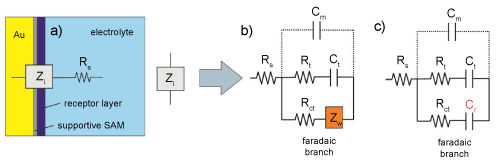Figure 11: a) Schematic representation of the interfacial impedance of the receptive interfaces used in biosensoring. b) The equivalent circuit capable of modelling impedimetric biosensor data where redox probe is present in solution phase. The Warburg element (Zw) accounts for the bulk diffusion characteristics of the redox probe and Rct is the redox charge transfer resistance. The electrolyte resistance (Rs) is modelled in series with the above total interfacial impedance (see (a)) and generally it is not important in the prevaling analysis once it does not vary. c) Is the appropriate equivalent circuit capable of modelling electrochemical capacitive biosensors. Note that interfacial capacitance of a monolayer dielectric modified electrode is defined by two series capacitances, those of the monolayer (Cm) and of the double-layer (Cdl) where Cdl>>Cm , meaning Cm dominates in analyses and is, therefore, the only capacitance represented in the equivalent circuit showed in these equivalent circuit models. Furthermore, Cm <